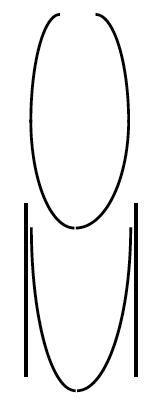### Home > INT3 > Chapter 2 > Lesson 2.2.2 > Problem2-68

2-68.

Think about how you might sketch a parabola.

1. Do the sides of a parabola ever curve in as in the figure at the right? Explain your reasoning.

No, because there is only one $y$-value for every $x$-value in a parabola.

2. Do the sides of the parabola approach straight vertical lines as shown in the figure at right? (In other words, do parabolas have vertical asymptotes?) Give a reason for your answer.

The domain of a parabola is all real numbers, so the graph must extend all the way left and right. Hence it never goes straight up.